Question

# You flip the same coin 90 mores times (100 total flips). If half of the 90...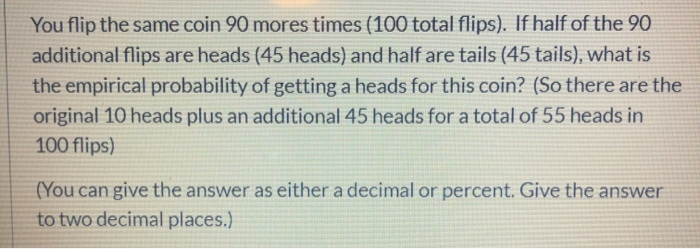You flip the same coin 90 mores times (100 total flips). If half of the 90 additional flips are heads (45 heads) and half are tails (45 tails), what is the empirical probability of getting a heads for this coin? (So there are the original 10 heads plus an additional 45 heads for a total of 55 heads in 100 flips) (You can give the answer as either a decimal or percent. Give the answer to two decimal places.)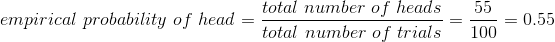#### Earn Coins

Coins can be redeemed for fabulous gifts.

Similar Homework Help Questions
• ### Flip a coin 10 times and record the observed number of heads and tails. For example,...

Flip a coin 10 times and record the observed number of heads and tails. For example, with 10 flips one might get 6 heads and 4 tails. Now, flip the coin another 20 times (so 30 times in total) and again, record the observed number of heads and tails. Finally, flip the coin another 70 times (so 100 times in total) and record your results again. We would expect that the distribution of heads and tails to be 50/50. How...

• ### You have a biased coin, where the probability of flipping a heads is 70%. You flip...

You have a biased coin, where the probability of flipping a heads is 70%. You flip once, and the coin comes up tails. What is the expected number of flips from that point (so counting that as flip #0) until the number of heads flipped in total equals the number of tails?

• ### 1. Multiple choice. Circle all the correct answers a) You flip a coin 100,000 times and...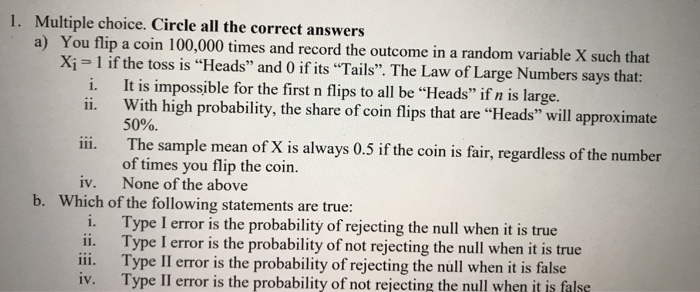1. Multiple choice. Circle all the correct answers a) You flip a coin 100,000 times and record the outcome in a Xi 1 if the toss is "Heads" and 0 if its "Tails. The Law of Large Numbers says that: i. ii. It is impossible for the first n flips to all be "Heads" if n is large. With high probability, the share of coin flips that are "Heads" will approximate 50%. The sample mean of X is always 0.5...

• ### Would you be more likely to get at least 70% tails if you flip a fair coin 10 times or if you flip a fair coin 1000 times

Would you be more likely to get at least 70% tails if you flip a fair coin 10 times or if you flip a faircoin 1000 times?A) You would be more likely to get at least 70% tails if you flip a fair coin 10 times than if youflip a fair coin 1000 times.B) The probability of getting at least 70% tails would be the same regardless of whether the coinis flipped 10 or 1000 times.C) You would be more...

• ### Exercise 1.16. We flip a fair coin five times

Exercise 1.16. We flip a fair coin five times. For every heads you pay me \$1 and for every tails I pay you \$1. Let X denote my net winnings at the end of five flips. Find the possible values and the probability mass function of X.

• ### Flip a fair coin 9 times

Flip a fair coin 9 times. Find the probability of getting the following outcome. (Round your answer to six decimal places.) at least 5 heads

• ### thank you !! 1. If you were to flip a coin 16 times. A) What is...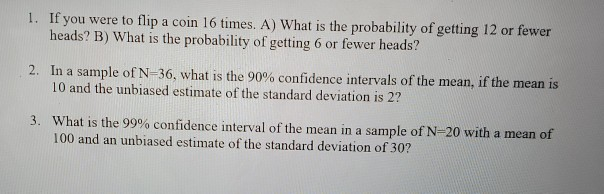thank you !! 1. If you were to flip a coin 16 times. A) What is the probability of getting 12 or fewer heads? B) What is the probability of getting 6 or fewer heads? 2. In a sample of N=36, what is the 90% confidence intervals of the mean, if the mean is 10 and the unbiased estimate of the standard deviation is 2? 3. What is the 99% confidence interval of the mean in a sample of N=20...

• ### If you flip a coin three times, what is the probability (represented in percent) of getting...

If you flip a coin three times, what is the probability (represented in percent) of getting three heads? Question 15 options: 0.13% 2.5% 12.5% 18.9%

• ### . Discrete Distributions. Suppose I flip a coin 40 times. The flips are independent. The probability...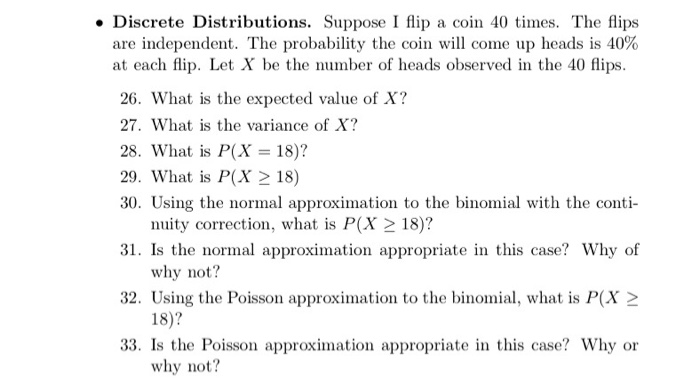. Discrete Distributions. Suppose I flip a coin 40 times. The flips are independent. The probability the coin will come up heads is 40% at each flip. Let X be the number of heads observed in the 40 flips. 26. What is the expected value of X? 27. What is the variance of X? 28. What is P(X 18)? 29. What is P(X 2 18) 30. Using the normal approximation to the binomial with the conti 31. Is the normal...

• ### PLEASE ANSWER QUESTION # 5 a and b !!!!!!!!!! 4) Chapter 4-5: a) You flip a balanced coin 3 times. What is the probabil...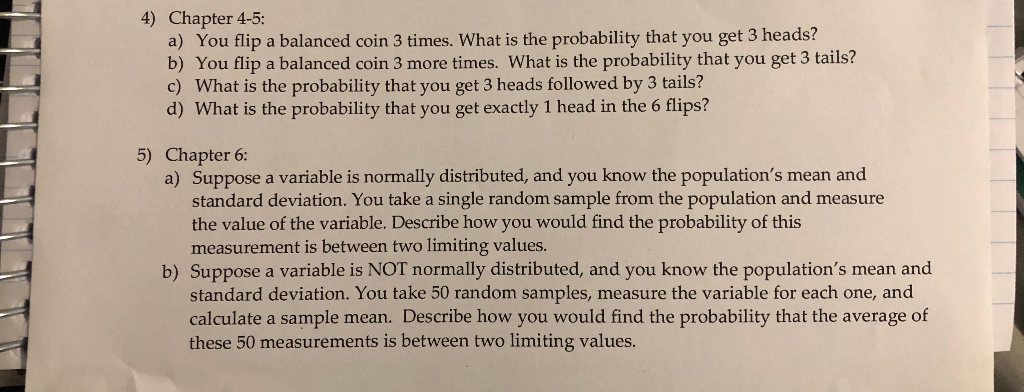PLEASE ANSWER QUESTION # 5 a and b !!!!!!!!!! 4) Chapter 4-5: a) You flip a balanced coin 3 times. What is the probability that you get 3 heads? b) You flip a balanced coin 3 more times. What is the probability that you get 3 tails? c) What is the probability that you get 3 heads followed by 3 tails? d) What is the probability that you get exactly 1 head in the 6 flips? 5) Chapter 6: a)...